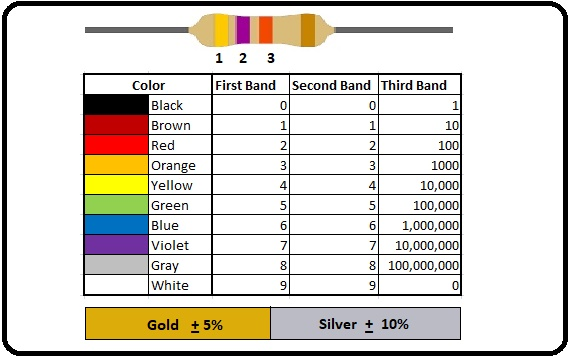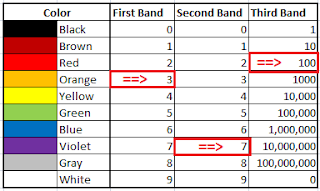basic idea about resistor its two pin electrical component that control flow of current or create resistance in current flow.

## Basic idea about resistor - What is resistor

Resistor is a two pin electrical component that control flow of current or create resistance in current flow. The different markings on the resistor represent different values.Resistor Color Code Chart

### How to read Resistor Color Code:

Each color has different value we calculate this value using Resistor Color Code Chart.

• The 1st and 2nd band represent resistor value.
• The 3rd band represent multiplier.
• And last 4th color gold and silver represent tolerance.Resistor
We learn resistor value calculation using this picture example.

In this picture Resistor has 4th color.
We know 1st, 2 color represent the resistor value so in color code chart see 1st color value in 1st band and 2nd color value in 2nd band.

• 1st color is orange in 1st band orange color value is 3Resistor color code find example
• 2nd color is violet in 2nd band violet color value is 7

mean 1st two color (orange and violet) value is 37

• 3rd color is red in 3rd band red color value is 100

we know in resistor 3rd color represent multiplier
so we multiply 1st 2 value (37) with 3rd value (100)
37 x 100 = 3700 ohm
mean this resistor value is 3700 ohm.

• 4th color is Gold we know its represent Torrance or actual value difference.
We can expect 5% plus and less of actual value (3700 ohm) of the resistor.
if we find 5% of 3700 so answer is 185.
So
185 ohm plus or less in actual value(3700 ohm).

#### The resistor has 2 sides witch side we start to calculate value?Two side of resistor
It's very simple every time when you calculate any resistor value make sure gold or silver color in your right side. (In picture very clear gold color on right side)
And start calculation with left to right.
Labels: